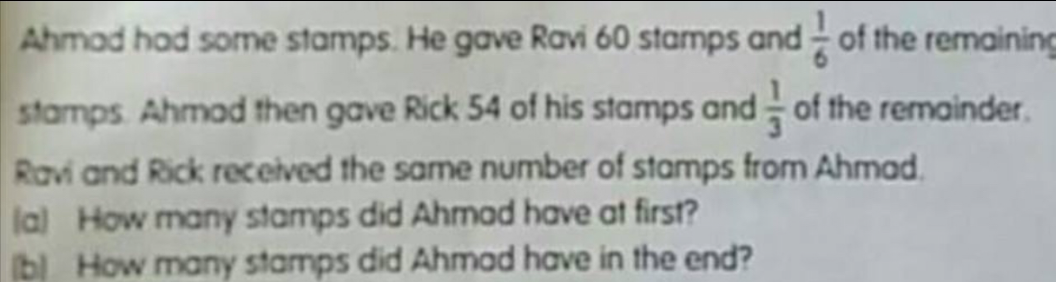# QuestionHi appreciate your help on this question using branching method thks

I know that you requested for branching method. However, I do not recommend that as it is overly algebraic. Therefore I posted an easier alternative below:

Hope you find this helpful.

All the best!

Subscribe to my YouTube channel for more Questions & Answers.

0 Replies 1 Like ✔Accepted Answer

This is a Branching question.

 Ravi Rick left total R1 60 + 1a 5a 6a  + 60 R2 54  + 1b 2b 3b + 54

60 + 1a = 54 + 1b

1a + 60 – 54 = 1b

1b = 1a + 6

3b = 3a + 18

3a + 18 + 54 = 3a + 72

3a + 72 = 5a

72 = 5a – 3a

2a = 72

1a = 72÷ 2 =36

a)

6a = 36 × 6 = 216

216 + 60 = 276

b)

1b = 36 + 6 = 42

3b = 3 × 42 = 126

126 + 54 = 180

0 Replies 0 Likes